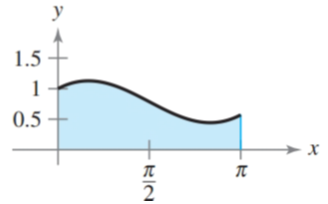Chapter 8.5, Problem 50E### Calculus: An Applied Approach (Min...

10th Edition
Ron Larson
ISBN: 9781305860919

#### Solutions

Chapter
Section### Calculus: An Applied Approach (Min...

10th Edition
Ron Larson
ISBN: 9781305860919
Textbook Problem
1 views

# Finding Area by Integration In Exercises 47-52, find the area of the region. See Example 7. y = x 2 + cos xTo determine

To calculate: The area bounded by the graph of y=x2+cosx.

Explanation

Given Information:

The provided graph of y=x2+cosx can be shown as:

Formula used:

The formula of definite integral of abcosudu.

abcosudu=[sinu]ab

The formula of definite integral of abundu.

abundu=[un+1n+1]ab

Calculation:

From provided diagram, the interval [a,b] will be [0, π]. Therefore, area bounded by graph of y=x2+cosx can be calculated as,

0π(x2+cosx)dx=0πx2dx+0πcosxdx=120πxdx+0πcosxdx

Integrate with the help of above integration formulae

### Still sussing out bartleby?

Check out a sample textbook solution.

See a sample solution

#### The Solution to Your Study Problems

Bartleby provides explanations to thousands of textbook problems written by our experts, many with advanced degrees!

Get Started

#### Differentiate the function. f(x) = e5

Single Variable Calculus: Early Transcendentals, Volume I

#### Solve the equation in Problem 1-6. 4.

Mathematical Applications for the Management, Life, and Social Sciences

#### Find the general indefinite integral. x54dx

Single Variable Calculus: Early Transcendentals

#### Simplify each expression. 24

Trigonometry (MindTap Course List)

#### True or False: is a convergent series.

Study Guide for Stewart's Multivariable Calculus, 8th

#### Distinguish between science and pseudoscience.

Research Methods for the Behavioral Sciences (MindTap Course List)